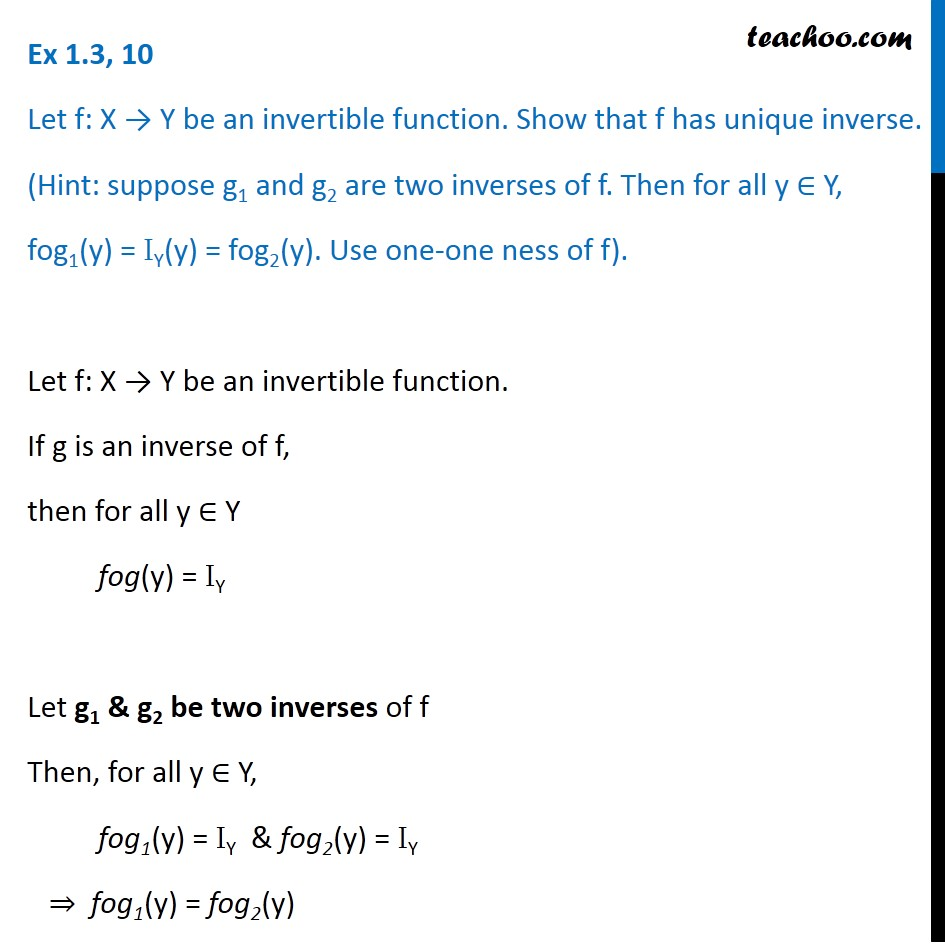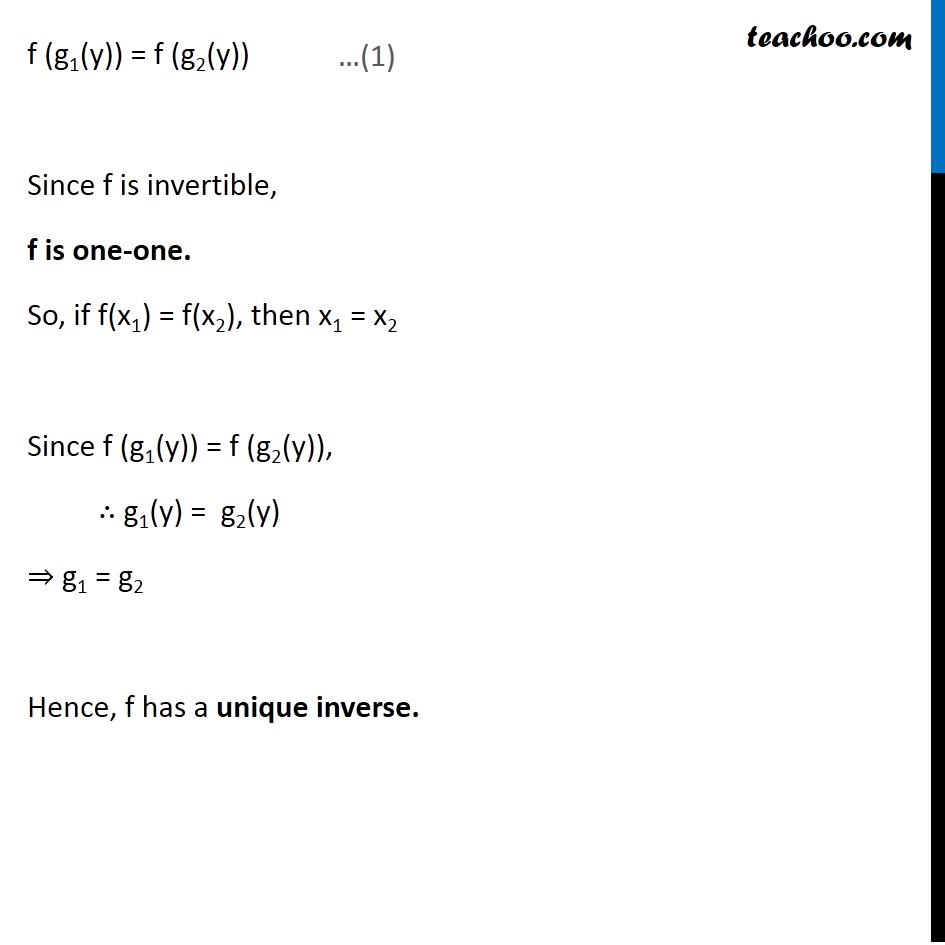Inverse of function: Proof questions

Chapter 1 Class 12 Relation and Functions
Concept wiseLearn in your speed, with individual attention - Teachoo Maths 1-on-1 Class

### Transcript

Ex 1.3, 10 Let f: X → Y be an invertible function. Show that f has unique inverse. (Hint: suppose g1 and g2 are two inverses of f. Then for all y ∈ Y, fog1(y) = IY(y) = fog2(y). Use one-one ness of f). Let f: X → Y be an invertible function. If g is an inverse of f, then for all y ∈ Y fog(y) = IY Let g1 & g2 be two inverses of f Then, for all y ∈ Y, fog1(y) = IY & fog2(y) = IY ⇒ fog1(y) = fog2(y) f (g1(y)) = f (g2(y)) Since f is invertible, f is one-one. So, if f(x1) = f(x2), then x1 = x2 Since f (g1(y)) = f (g2(y)), ∴ g1(y) = g2(y) ⇒ g1 = g2 Hence, f has a unique inverse.